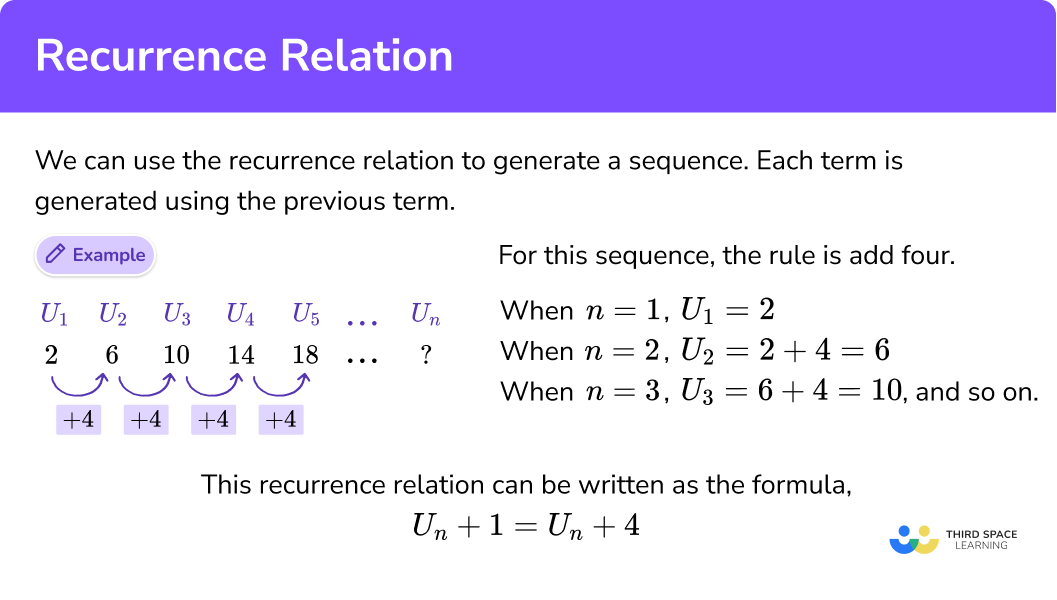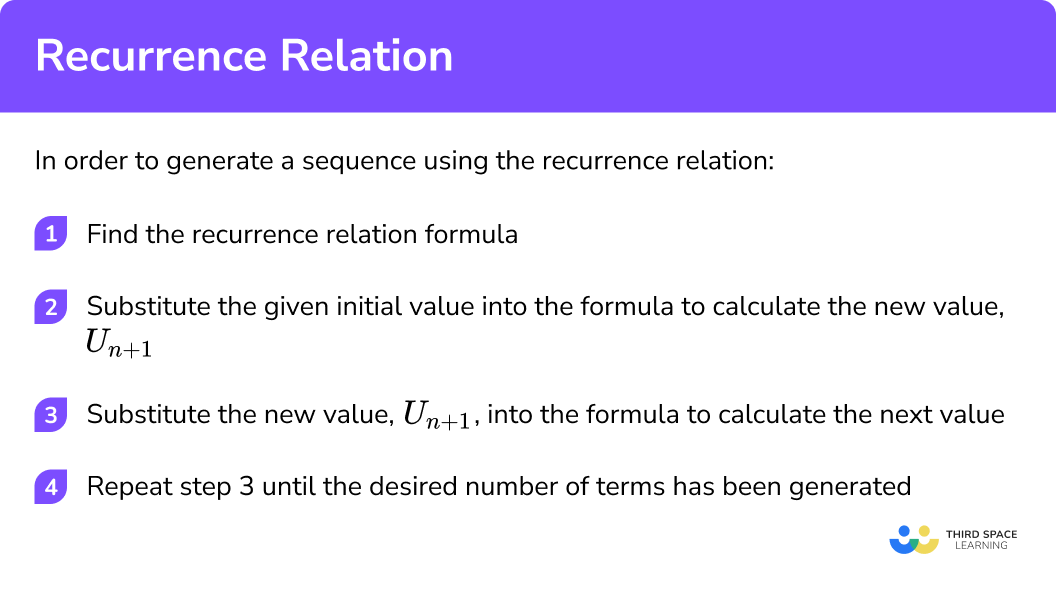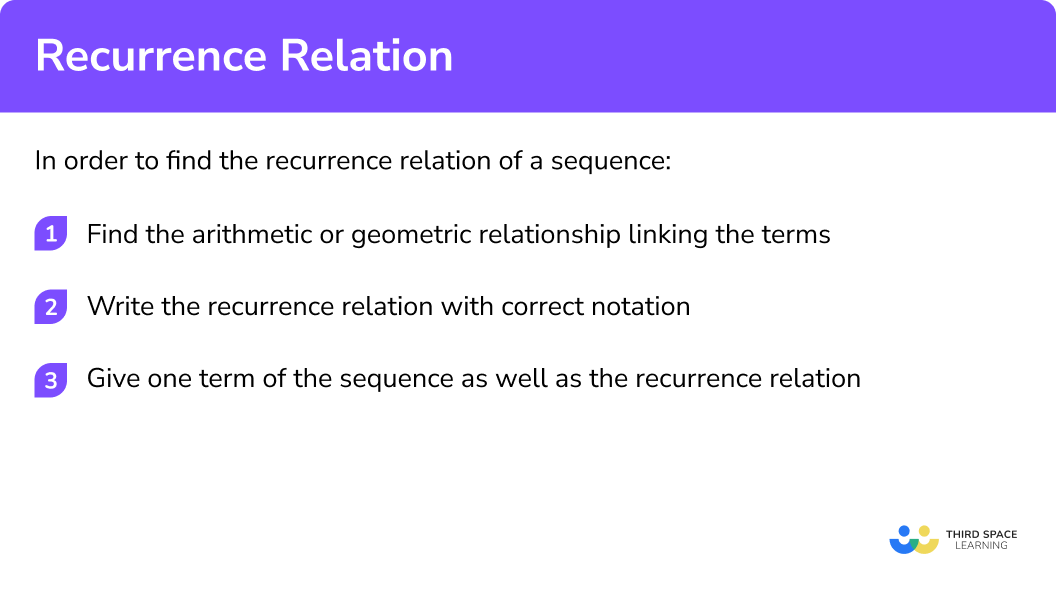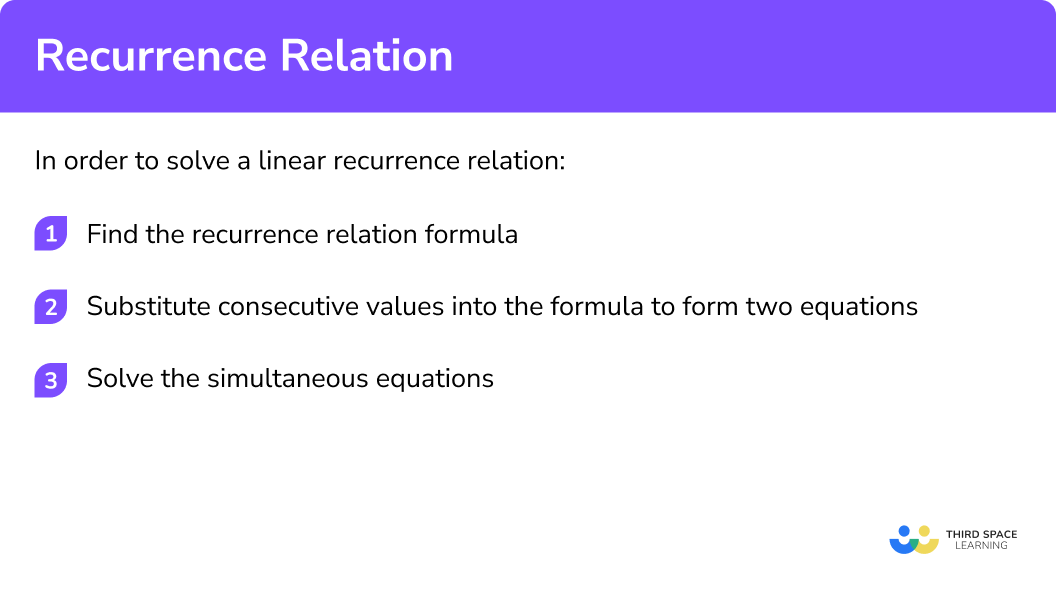GCSE Maths Algebra Sequences

Recurrence Relation

# Recurrence Relation

Here we will learn about recurrence relations, including generating sequences using the recurrence relation, finding the recurrence relation of a sequence and solving recurrence relations.

There are also recurrence relation worksheets based on Edexcel, AQA and OCR exam questions, along with further guidance on where to go next if you’re still stuck.

## What is the recurrence relation?

The recurrence relation is an equation that uses recursion to relate terms in a sequence. Recursion uses a rule over and over again. This relationship can be used to find the next term or previous terms, missing coefficients and its limit. This can also be seen in GCSE mathematics when working with iteration.

For example,

For this sequence, the rule is add four.

Each number in a sequence is called a term, and each term is identified by its position within the sequence. We can write them as,

The first term is U_1=2 .

The second term is U_2=6 .

The third term is U_3=10 .

The fourth term is U_4=14 .

The fifth term is U_5=18 .

The n th term is U_n .

To generate this sequence we can use the \textbf{n} th term formula for the sequence. For this sequence, the n th term would be U_n=4 n-3.

When n=1, \ U_1=4(1)-2=2.

When n=2, \ U_2=4(2)-2=6, and so on.

Step-by-step guide: Nth term of a sequence

Another way of generating this sequence would be to use the recurrence relation, where each term is generated using the previous value. These recurrence relations are algorithms and can generate any term in the sequence.

When n=1, \ U_1=2.

When n=2, \ U_2=2+4=6.

When n=3, \ U_3=6+4=10, and so on.

Hence, the recurrence equation can be written as the formula

U_{n+1}=U_n+4.

The initial value, U_1, would need to be provided. The initial value could also be U_0.

Recurrence relations can also be used to find particular solutions.

### What is the recurrence relation?### Fibonacci sequence

The following sequence of numbers is known as the Fibonacci numbers,

1, \ 1, \ 2, \ 3, \ 5, \ 8, \ 13, \ 21, …

The next number of a sequence can be found by adding the two previous terms together, therefore the Fibonacci numbers can be written using a recursion relationship. We need to be given the first 2 numbers in the Fibonacci sequence.

U_{n+1}=U_{n-1}+U_n, \ U_1=1, \ U_2=1

If we change the first 2 values we can generate a different Fibonacci sequence.

For example,

U_{n+1}=U_{n-1}+U_n, \ U_1=2, \ U_2=5

would give the sequence

2, \ 5, \ 7, \ 12, \ 19, \ 31, …

In general a Fibonacci sequence can be written as,

U_{n+1}=U_{n-1}+U_n, \ U_1=a, \ U_2=b

and would give the sequence

a, \ b, \ a+b, \ a+2b, \ 2a+3b, \ 3a+5b, …

Did you know the Fibonnaci numbers occur often in nature? Flowers often have 3 or 5 or 8 petals. This is why a clover with 4 petals is difficult to find and is considered lucky.

## How to generate a sequence using the recurrence relation

In order to generate a sequence using the recurrence relation:

1. Find the recurrence relation formula.
2. Substitute the given initial value into the formula to calculate the new value, U_{n+1}.
3. Substitute the new value, U_{n+1}, into the formula to calculate the next value.
4. Repeat step 3 until the desired number of terms has been generated.

### How to generate a sequence using the recurrence relation### Related lessons on sequences

Recurrence relation is part of our series of lessons to support revision on sequences. You may find it helpful to start with the main sequences lesson for a summary of what to expect, or use the step by step guides below for further detail on individual topics. Other lessons in this series include:

## Generating sequences using the recurrence relation examples

### Example 1: generating a linear sequence

A sequence is defined by the recurrence relation U_{n+1}=5 U_n and has U_0=1.

Find the first four terms of the sequence.

1.  Find the recurrence relation formula.

The recurrence relation formula is given in the question, U_{n+1}=5 U_n.

2Substitute the given initial value into the formula to calculate the new value, U_{n+1}.

The initial value is given in the question, U_0=1.

Substituting this into the recurrence relation formula gives U_1=5 U_0=5 \times 1=5.

3Substitute the new value, U_{n+1}, into the formula to calculate the next value.

U_2=5 U_1=5 \times 5=25

4Repeat step 3 until the desired number of terms has been generated.

\begin{aligned} &U_3=5 U_2=5 \times 25=125 \\\\ &U_4=5 U_3=5 \times 125=625 \end{aligned}

Hence, the next four terms of the sequence are 5, \ 25, \ 125, \ 625.

### Example 2: generating a linear sequence

A sequence is given by the recurrence relation U_{n+1}=2 U_n+7.

If U_0=-2 find the next 5 terms.

The recurrence relation formula is given in the question, U_{n+1}=2 U_n+7 .

The initial value is given in the question, U_0=-2.

Substituting this into the recurrence relation formula gives

U_1=2 U_0+7=(2 \times-2)+7=-4+7=3.

U_2=2 U_1+7=(2 \times 3)+7=6+7=13

\begin{aligned} &U_3=2 U_2+7=(2 \times 13)+7=26+7=33 \\\\ &U_4=2 U_3+7=(2 \times 33)+7=66+7=73 \\\\ &U_5=2 U_4+7=(2 \times 73)+7=146+7=153 \end{aligned}

Hence, the next five terms of the sequence are 3, \ 13, \ 33, \ 73, \ 153.

### Example 3: generating a sequence for a polynomial

Find the first four terms of the sequence in which \ U_1=2 \ and \ U_{n+1}=U_n^2 \ for \ n ≥ 1.

The recurrence relation formula is given in the question, U_{n+1}=U_n^2.

The initial value is given in the question, U_1=2.

Substituting this into the recurrence relation formula gives

U_2=U_1^2=2^2=4.

U_3=U_2^2=4^2=16

U_4=U_3^2=16^2=256

Hence, the first four terms of the sequence are 2, \ 4, \ 16, \ 256.

## How to find the recurrence relation of a sequence

In order to find the recurrence relation of a sequence:

1. Find the arithmetic or geometric relationship linking the terms.
2. Write the recurrence relation with correct notation.
3. Give one term of the sequence as well as the recurrence relation.

### How to find the recurrence relation of a sequence## Finding the recurrence relation of a sequence examples

### Example 4: finding the recurrence relation of a linear sequence

Find the recurrence relation that satisfies the sequence 5, \ 8, \ 11, \ 14, \ 17, …

Each term in this sequence equals the one before it, plus 3.

The recurrence relation is written as U_{n+1}=U_n+3.

The description needs to be more specific, so giving one term in the sequence, as well as the recurrence relation is written as

U_{n+1}=U_n+3, \ U_1=5.

### Example 5: finding the recurrence relation of a linear sequence

Write down a recurrence relation which produces the sequence 3, \ 6, \ 12, \ 24, \ 48, …

Each term in this sequence is double the previous term.

The recurrence relation is written as U_{n+1}=2 U_n.

The description needs to be more specific, so giving one term in the sequence, as well as the recurrence relation is written as

U_{n+1}=2 U_{n}, \ U_1=3.

### Example 6: finding the recurrence relation of a linear sequence

Describe the sequence 32, 37, 42, 47, … using a recurrence relation.

Each term in this sequence equals the one before it, plus 5.

The recurrence relation is written as U_{n+1}=U_n+5.

The description needs to be more specific, so giving one term in the sequence, as well as the recurrence relation is written as

U_{n+1}=U_n+5, \ U_1=32.

## How to solve a linear recurrence relation

In order to solve a linear recurrence relation:

1. Find the recurrence relation formula.
2. Substitute consecutive values into the formula to form two equations.
3. Solve the simultaneous equations.

### How to solve a linear recurrence relation## Solving linear recurrence relation examples

### Example 7: solving a linear recurrence relation

In a sequence U_1=2, \ U_2=8 and U_3=26. If the recurrence relation is of the form U_{n+1}=a U_n+b, find the values of the constants a and b.

The recurrence relation formula is given in the question, U_{n+1}=a U_n+b.

Using U_1=2, \ U_2=8,

\begin{aligned} &U_2=a U_1+b \\\\ &8=2 a+b. \end{aligned}

Using U_2=8, \ U_3=26,

\begin{aligned} &U_3=a U_2+b \\\\ &26=8 a+b. \end{aligned}

The simultaneous equations to be solved are

\begin{aligned} &2 a+b=8 \\\\ &8 a+b=26 \end{aligned}

Eliminating b, and solving for a, gives

\begin{aligned} &6 a=18 \\\\ &a=3 \end{aligned}

Substituting a=3, into one of the equations and solving for b, gives

\begin{aligned} &6+b=8 \\\\ &b=2 \end{aligned}

Hence, a=3 and b=2.

### Example 8: solving a linear recurrence relation

A sequence is defined by the recurrence relation U_n=a U_{n-1}+b. Find the values of a and b if U_1=-3, \ U_2=7 and U_3=10.

The recurrence relation formula is given in the question, U_n=a U_{n-1}+b.

Note, in this example the recurrence relation contains the notation U_{n-1} not U_{n+1}.

This is important when substituting consecutive values to form equations to be solved.

Using U_1=-3, \ U_2=7,

\begin{aligned} &U_2=a U_1+b \\\\ &7=-3 a+b. \end{aligned}

Using U_2=7, \ U_3=10,

\begin{aligned} &U_3=a U_2+b \\\\ &10=7 a+b. \end{aligned}

The simultaneous equations to be solved are

\begin{aligned} &-3 a+b=7 \\\\ &7 a+b=10 \end{aligned}

Eliminating b, and solving for a, gives

\begin{aligned} &10 a=3 \\\\ &a=0.3 \end{aligned}

Substituting a=0.3, into one of the equations and solving for b, gives

\begin{aligned} &7 a+b=10 \\\\ &2.1+b=10 \\\\ &b=7.9 \end{aligned}

Hence, a=0.3 and b=7.9

### Example 9: solving a linear recurrence relation

The sequence 20, \ 15, \ 12.5, … has a recurrence relation U_{n+1}=a U_n+b. Calculate a and b.

The recurrence relation formula is given in the question, U_{n+1}=a U_n+b.

The question does not state the values to be used at this step, however, we can identify them from the sequence, in this instance, U_1=20, \ U_2=15 and U_3=12.5.

Using U_1=20, \ U_2=15,

\begin{aligned} &U_2=a U_1+b \\\\ &15=20 a+b. \end{aligned}

Using U_2=15, \ U_3=12.5,

\begin{aligned} &U_3=a U_2+b \\\\ &12.5=15 a+b. \end{aligned}

The simultaneous equations to be solved are

\begin{aligned} &20 a+b=15 \\\\ &15 a+b=12.5 \end{aligned}

Eliminating b, and solving for a, gives

\begin{aligned} &5 a=2.5 \\\\ &a=0.5 \end{aligned}

Substituting a=0.5, into one of the equations and solving for b, gives

\begin{aligned} &20 a+b=15 \\\\ &10+b=15 \\\\ &b=5 \end{aligned}

Hence, a=0.5 and b=5.

### Common misconceptions

• Recurrence notation

It can be easy to misunderstand the recurrence notation.

U_1 is the first term, U_2 is the second term, and so on.

U_n is the nth term, U_{n+1} is the next term, and U_{n-1} is the previous term.

So a sequence looks like,

U_1, \ U_2, … , \ U_{n-1}, \ U_n, \ U_{n+1}, …

### Practice recurrence relation questions

1. Describe the sequence 1, \ 6, \ 11, \ 16, \ 21, … using a recurrence relation.

U_{n+1}=U_n+5, \ U_1=1U_n=U_{n+1}+5U_{n+1}=5 U_nU_{n+1}=U_n+5Each term in this sequence equals the one before it, plus 5.

The recurrence relation can be written as U_{n+1}=U_n+5.

However, we need to be more specific, so giving one term in the sequence, as well as the recurrence relation is required for the description.

U_{n+1}=U_n+5, \ U_1=1

2. Find the first 5 terms of the sequence in which U_1=7 and U_{n+1}=U_n+3.

3, \ 6, \ 9, \ 12, \ 15, …7, \ 10, \ 13, \ 16, \ 19, …7, \ 21, \ 63, \ 189, \ 567, …7, \ 4, \ 1, \ -2, \ -5, …The first term, U_1=7.

The recurrence relation, U_{n+1}=U_n+3, tells us we add 3 to the previous term, U_n to get the next term, U_{n+1}.

Giving the sequence, 7, \ 10, \ 13, \ 16, \ 19, …

3. Describe the sequence 34, \ 30, \ 26, \ 22, \ 18, … using a recurrence relation.

U_n=U_{n+1}-4U_{n+1}=4 U_nU_{n+1}=U_n+4, \ U_1=34U_{n+1}=U_n-4, \ U_1=34Each term in this sequence equals the one before it, minus 4.

The recurrence relation can be written as U_{n+1}=U_n-4.

However, we need to be more specific, so giving one term in the sequence, as well as the recurrence relation is required for the description.

U_{n+1}=U_n-4, \ U_1=34

4. Find the next 5 terms of the sequence in which U_0=44 and U_{n+1}=U_n-2.

44, \ 42, \ 40, \ 38, \ 36, …44, \ 46, \ 48, \ 50, \ 52, …42, \ 40, \ 38, \ 36, \ 34, …42, \ 44, \ 46, \ 48, \ 50, …The recurrence relation, U_{n+1}=U_n-2, tells us we subtract 2 from the previous term, U_n to get the next term, U_{n+1}.

The sequence can be continued by subtracting 2.

U_1=U_0-2=44-2=42

Giving the sequence, 42, \ 40, \ 38, \ 36, \ 34, …

5. Describe the sequence 4, \ 8, \ 16, \ 32, \ 64, … using a recurrence relation.

U_n=2 U_{n+1}U_{n+1}=2 U_{n}, \ U_1=4U_{n+1}=2 U_nU_{n+1}=\frac{1}{2} U_nEach term in this sequence is double the previous term.

The recurrence relation can be written as U_{n+1}=2 U_n.

However, we need to be more specific, so giving one term in the sequence, as well as the recurrence relation is required for the description.

U_{n+1}=2 U_n, \ U_1=4

6. A sequence is defined by the recurrence relation U_{n+1}=a U_n+b. Find the values of a and b if U_1=5, \ U_2=27 and U_3=137.

a=1.375, \ b=5.125a=5.125, \ b=1.375a=2, \ b=5a=5,\ b=2Substituting pairs of consecutive values to form simultaneous equations.

Using U_1=5, \ U_2=27,

\begin{aligned} &U_2=a U_1+b \\\\ &27=5 a+b. \end{aligned}

Using U_2=27, \ U_3=137,

\begin{aligned} &U_3=a U_2+b \\\\ &137=27 a+b. \end{aligned}

Solving them gives a=5 and b=2.

### Recurrence relation GCSE questions

1. Find the next three terms of a sequence with recurrence relation U_{n+1}=2U_n+5, \ U_1=3.

(3 marks)

Substituting U_1 to get U_2.

For example, 2U_1+5=(2 \times 3)+5=11.

(1)

Substituting U_2 to get U_3.

For example, 2U_2+5=(2 \times 11)+5=27.

(1)

Substituting U_3 to get U_4.

For example, 2U_3+5=(2 \times 27)+5=59.

(1)

2. Describe the sequence 3, \ 8, \ 13, \ 18, \ 23, …

using a recurrence relation.

U_{n+1}=U_n+d, \ U_1=a.

(3 marks)

Identifying the common difference, for example, 5.

(1)

Writing the recurrence relation, U_{n+1}=U_n+5.

(1)

Writing the recurrence relation and one term from the sequence

U_{n+1} = U_n +5, \ U_1 =3 .

(1)

3. A sequence is defined by the recurrence relation U_{n+1} = aU_n +b.

Find the values of a and b if U_1=4, \ U_2=9 and U_3=24. \ a and b are integers.

(4 marks)

Substitute a pair of consecutive values to form an equation,

9=4a+b, or 24=9a+b.

(1)

Forming both equations,

9=4a+b and 24=9a+b.

(1)

Eliminating one variable and solving for the other,

a=3 or b=-3.

(1)

Solving and getting a=3 and b=-3.

(1)

## Learning checklist

You have now learned how to:

• Generate a sequence from a recurrence relation
• Find a recurrence relation for a sequence
• Solve a recurrence relation

## Recurrence relations at A Level

Recurrence relations and recursive algorithms feature heavily in A Level mathematics when working with characteristic equations of first order linear differential equations (difference equations) and higher order recurrence relations.

## Still stuck?

Prepare your KS4 students for maths GCSEs success with Third Space Learning. Weekly online one to one GCSE maths revision lessons delivered by expert maths tutors.

Find out more about our GCSE maths tuition programme.